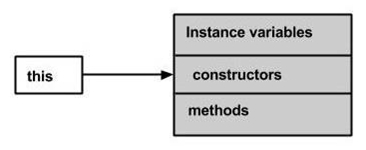# When should I use the keyword ‚Äòthis‚Äô in a Java class?

The this is a keyword in Java which is used as a reference to the object of the current class, within an instance method or a constructor. Using this you can refer the members of a class such as constructors, variables, and methods.## Example

Live Demo

public class This_Example {
// Instance variable num
int num = 10;

This_Example() {
System.out.println("This is an example program on keyword this");
}
This_Example(int num) {

// Invoking the default constructor
this();

// Assigning the local variable num to the instance variable num
this.num = num;
}
public void greet() {
System.out.println("Hi Welcome to Tutorialspoint");
}
public void print() {
// Local variable num
int num = 20;

// Printing the local variable
System.out.println("value of local variable num is : "+num);

// Printing the instance variable
System.out.println("value of instance variable num is : "+this.num);

// Invoking the greet method of a class
this.greet();
}
public static void main(String[] args) {
// Instantiating the class
This_Example obj1 = new This_Example();

// Invoking the print method
obj1.print();

// Passing a new value to the num variable through parametrized constructor
This_Example obj2 = new This_Example(30);

// Invoking the print method again
obj2.print();
}
}


## Output

This is an example program on keyword this
value of local variable num is : 20
value of instance variable num is : 10
Hi Welcome to Tutorialspoint
This is an example program on keyword this
value of local variable num is : 20
value of instance variable num is : 30
Hi Welcome to Tutorialspoint